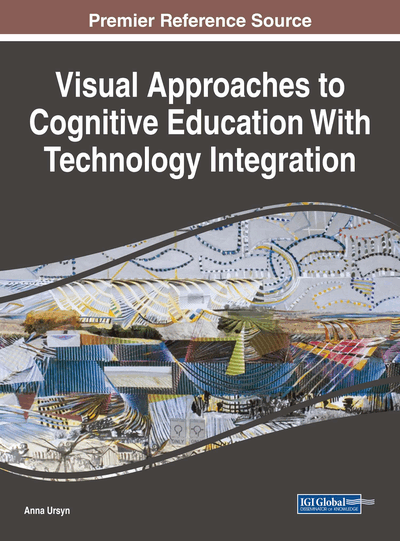# The Four-Color Theorem and the Geometry of Nature

Jean Constant (Hermay.org., USA)
DOI: 10.4018/978-1-5225-5332-8.ch004

## Abstract

The four-color theorem stands at the intersection of Science and Art. The mathematical reasoning used to solve the theorem lead to many practical applications in mathematics, graph theory, and computer science. One aspect of the four-color theorem, which was seldom covered and relevant to the field of visual communication, is the actual effectiveness of the distinct 4 colors scheme chosen to define its mapping. This chapter briefly reviews the historical and scientific background of the theorem, puts in perspective contemporary scientific and technical data pertaining to the perception of color, and studies the theorem in a particular color scheme on a fluorite crystal, which was chosen because of its colorless appearance and the mathematically symmetrical nature of its structure.
Chapter Preview
Top

## Introduction

The four-color theorem states that any map in a plane can be colored using four colors in such a way that regions sharing a common boundary (other than a single point) do not share the same color. – Eric Weisstein, “The Four-Color Theorem.” Wolfram Mathematica. Wolfram MathWorld (2017).

Eric Weisstein (2017a) discussed this theory with respect to the map coloring. Although German mathematician Klein briefly referred to the concept earlier in a public lecture, most agree that the student at the London University College London Francis Guthrie originated the question that became the four-color problem in 1852 (O'Connor & Robertson, 1996). His conjecture quickly caught the attention of many prominent mathematicians of the time: Augustus De Morgan, Arthur Cayley in 1878, Alfred Kempe in1879, Tait in 1880, and Percy John Heawood among many. It was finally solved by Karel Appel and Wolfgang Haken in 1977. They wrote a computer-assisted proof that demonstrated that four colors were sufficient. The proof required a thousand hours of computer time, a hundred pages of summary, massive amount of detailed instructions, and seven hundred pages of backup work. The computer printouts for it stacked to four feet high (Wilson, 2003). A year later, using a separate procedure F. Allaire of the University of Waterloo, Ontario, CA, demonstrated the theorem in just 50 hours of human-computer dialogue (Popescu, & Mastorakis, 2009). In 1996, Robertson et al. proposed a shorter, independent proof that G. Gonthier and B. Werner confirmed in 2004. However, because part of the proof consisted of an exhaustive analysis of many discrete cases by a computer, some mathematicians do not accept it (Weisstein, 2017b). The original finding proposed 1,936 configurations. Later 102 of them were found redundant, thus reducing the number of configurations to 1,834, a number later reduced to 1,482. Verifying a computer program may be as challenging as checking a mathematical proof of the same length.

The theorem reasoning has benefited many mathematical fields of research: problem-solving, graph theory, networking; computer science, and communications networks among other. Guthrie’s conjecture was also anchored on a practical issue having to do with visualization best practices – the procedures that carry a visual signal most effectively. The practical representation of the theorem was initially based on a unique four-color scheme (red, yellow, blue, and green) that has been used consistently since (Constantinou & Ampatzoglou, 2007). Even today, we know very little of the process that led to this particular choice of color. The mathematical proof of theorem relied on the concept of reducibility. What lead the mathematicians involved in solving the problem to choose green over any other color of the spectrum? In that context, was green the most effective choice for a four color scheme and if so, can the field of visual communication benefit from the theorem in the same manner the mathematical field did? All these significant questions legitimate the dialogue between the science and art disciplines and could help progress both further in their own research.

## Complete Chapter List

Search this Book:
Reset